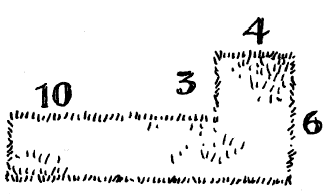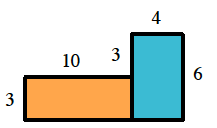### Home > CC2 > Chapter 4 > Lesson 4.1.2 > Problem4-17

4-17.

While Mrs. Poppington was visiting the historic battleground at Gettysburg, she talked with the landscapers who were replacing the sod in a park near the visitor center. The part of the lawn being replaced is shown in the diagram at right. Measurements are in yards. The diagram is not drawn to scale, but all corners are right angles.1. Use your ruler to make a scale drawing of the lawn that is being replaced. Let $\frac { 1 } { 4 }$ inch equal $1$ yard.

Draw the diagram on your paper. Be sure to scale it correctly.

2. How many square yards of sod will be needed?

Find the area of the diagram in square yards.

Split the diagram into two rectangles. Find the area of each rectangle respectively and add them together to find the total area.

$\text{Total area} =3(10)+4(6)$
$\text{Total area}=54\text{ square yards}$3. At $\2.43$ a square yard, how much will the sod cost?

Multiply the cost per square yard by the total square yards of sod needed.

4. The area will be surrounded by a temporary fence which costs $\0.25$ per linear foot. How many feet will be needed, and how much will it cost?

Find the perimeter of the diagram to find how many feet will be needed and multiply that by \$0.25 to find how much it will cost.

5. What will be the total cost of materials for the sod and fencing?